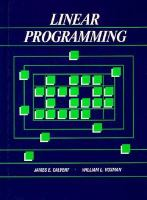Close

# Linear Programming

## by James Calvert• ISBN: 9780155510272
• ISBN10: 0155510274

# Linear Programming

## by James Calvert

• List Price: \$353.95
• Binding: Hardcover
• Edition: 01
• Publisher: Cengage
• Publish date: 03/01/1989
• ISBN: 9780155510272
• ISBN10: 0155510274
used \$137.01
You save: 61%
Description: 1. LINEAR PROGRAMMING: AN OVERVIEW AND SAMPLE PROBLEMS. Product-mix Problems. Transportation and Assignment Problems. General Comments Regarding LP Models. Dynamic Planning. 2. GEOMETRIC AND ALGEBRAIC PRELIMINARIES. Half-spaces, Hyperplanes, and Convex Sets. Feasible Sets and Geometric Solutions of LP Problems. Proof of the Corner Point Theorem. Algebraic Preliminaries. Slack Variables and Constraints in Canonical Form. 3. THE SIMPLEX METHOD. Selection of Entering and Departing Basic Variables. Simplex Tableaus. Degeneracy, Cycling, and Convergence. The Two-Phase Method. The Two-Phase Method: Some Complications. The Big-M Method. LP Models. 4. THE SIMPLEX METHOD IN A MATRIX CONTEXT. Matrices. Elementary Matrices, Matrix Inverses, and Pivoting. Matrix Representation of the Simplex Method: Part I. Matrix Representation of the Simplex Method: Part II. LP Models. 5. THE REVISED SIMPLEX METHOD. A Faster Algorithm for Computer Solution. Preliminary Results for Use in Complexity Analysis. Space and Time Complexity of the Simplex Method. LP Models. 6. DUALITY. An Economic Interpretation of Duality. Primal and Dual Problems: A Special Case. Duality: The General Case. Duality Proofs. The Dual Simplex Model. LP Models. 7. SENSITIVITY ANALYSIS. What is Sensitivity Analysis? Changes in the Resource Column. Changes in Cost Coefficients. Using Duality in Sensitivity Analysis. Introduction of a New Variable. LP Models. 8. AN INTRODUCTION TO INTEGER PROGRAMMING. A Sampling of IP Problems. A Branch and Bound Method. Implicit Enumeration: Part I. Implicit Enumeration: Part II. Geometry Cutting Plane Method. LP Models. 9. THE TRANSPORTATION PROBLEM. Choosing the Entering Basic Variable. Choosing the Departing Basic Variable. Finding an Initial Basic Feasible Solution. 10. LARGE-SCALE PROBLEMS. LP Problems with Bounded Constraints. A Decomposition Procedure: The Master Problem. Solving the Master Problem. Final Words.
Expand description